The boundary-layer theory (2) -temperature beneath the plate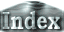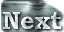In light of the schematic picture above, we define the temperature at the bottom of the plate to be Tp, the temperature of the fluid below the plate to be Tcp, and the temperature of the internal fluid outside the plate to be Tc. Since the temperature of the fluid below the plate Tcp is specified by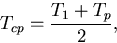(5)
it is sufficient to evaluate Tp in order to obtain Tcp.

Using boundary-layer theory (Turcotte and Schubert 1982), the relation between the Nusselt number and the Rayleigh number is given by(6)
where the prime denotes values for the convection under the plate, and a is the horizontal size of a convection cell. Since temperature and its gradient must be continuous at the boundary, we have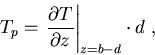(7)
For the fluid beneath the plate, the thickness of the layer is 1-d and temperature at the top of the fluid layer is the temperature just beneath the plate Tp, Ra' is expressed in terms of the Rayleigh number for the whole layer Ra as,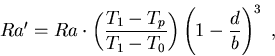(8)
From these equations, a relation between the temperature under the plate Tp and the Rayleigh number Ra can be straightforwardly obtained.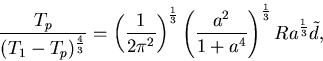(9)
From (9), Tp can be calculated for a given value of the Rayleigh number. Further, the averaged temperature of the fluid under the plate Tcp = (T1+Tp)/2 and temperature difference induced by the plate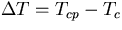can also be estimated.

 The boundary-layer theory (2) -temperature beneath the plate